# Introductory examples¶

Introductory examples for time series in general.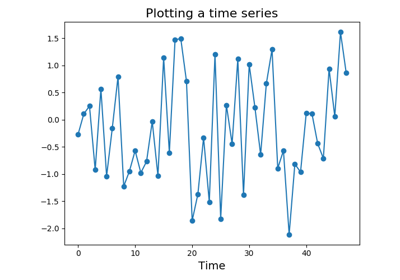Plotting a time series

# Approximating time series¶

Approximation algorithms try to capture the most important information from time series. They can be seen as simple feature extraction algorithms.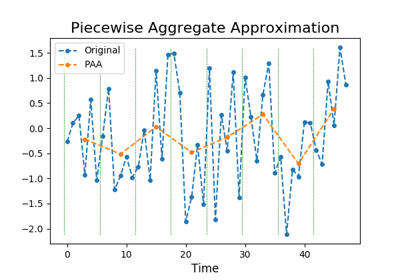Piecewise Aggregate Approximation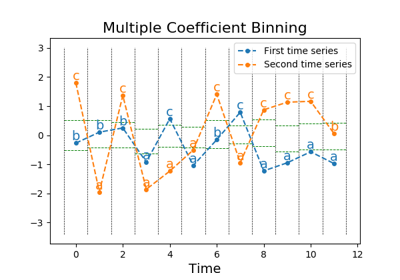Multiple Coefficient Binning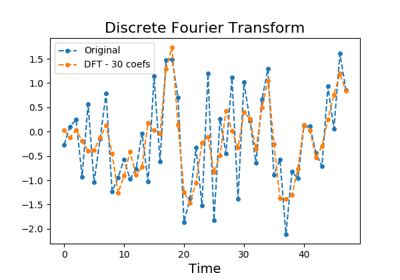Discrete Fourier Transform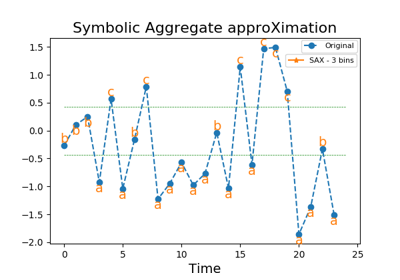Symbolic Aggregate approXimation

# Bag-of-words transformation¶

Bag-of-words algorithms transform a sequence of symbols into a bag of words.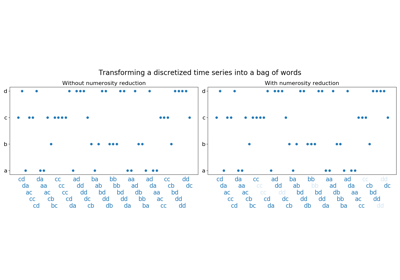Bag of Words

# Classification algorithms¶

Classification algorithms can directly classify raw time series.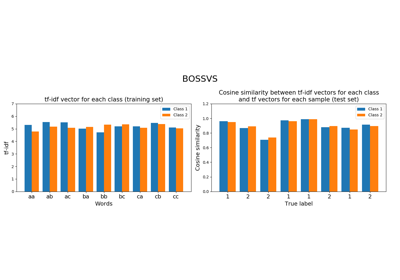Bag-of-SFA Symbols in Vector Space (BOSSVS)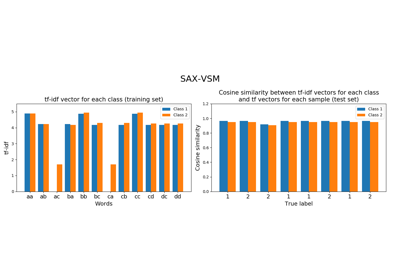Symbolic Aggregate approXimation in Vector Space Model (SAX-VSM)

# Dataset utilities¶

Examples on how to load and make time series datasets.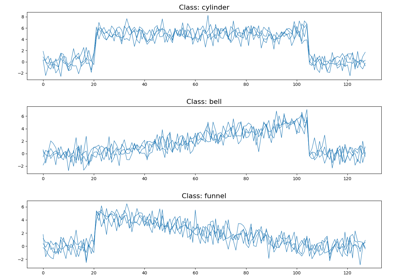Making a Cylinder-Bell-Funnel dataset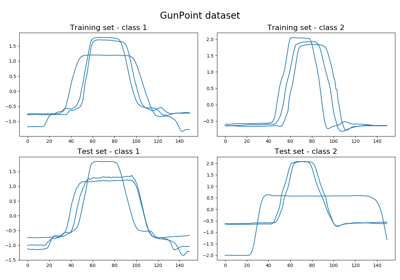# Decomposing time series¶

Decomposition algorithms decompose time series into several time series.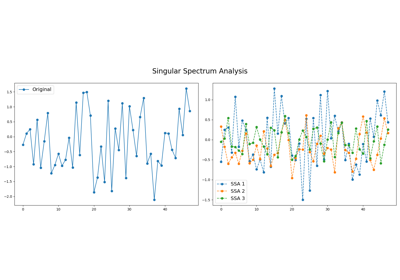Singular Spectrum Analysis

# Imaging time series¶

Imaging algorithms transform time series into images.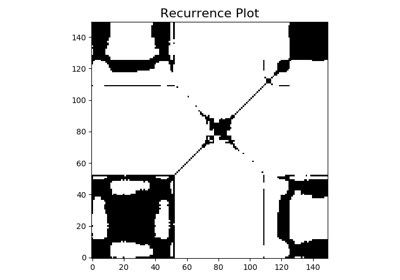Recurrence Plot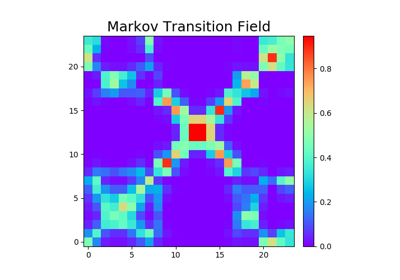Markov Transition Field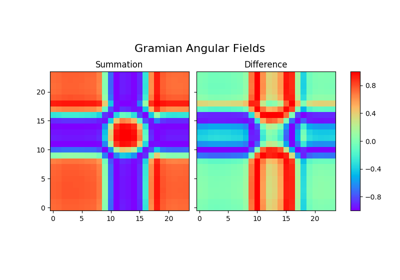Gramian Angular Field

# Metrics¶

Specific metrics for time series have been developed. The examples below illustrate some of the implemented metrics.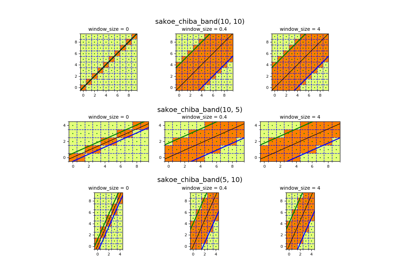Sakoe-Chiba band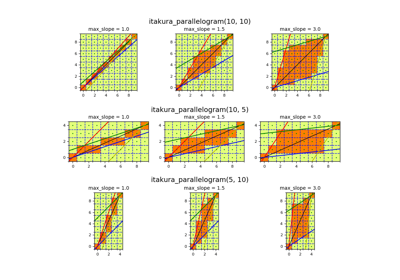Itakura parallelogram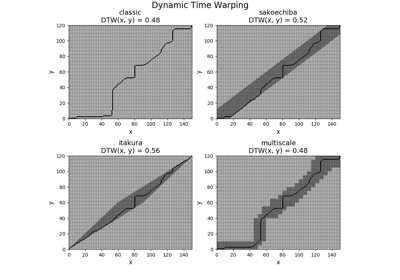Dynamic Time Warping

# Multivariate time series¶

Specific algorithms for multivariate time series have been developed. The examples below illustrate some of the implemented ones.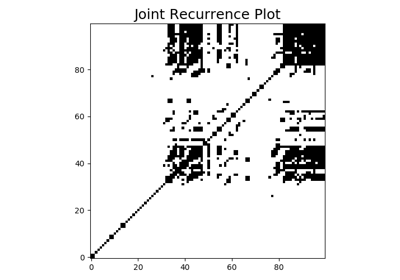Joint Recurrence Plot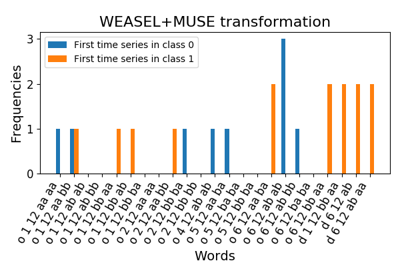WEASEL+MUSE

# Preprocessing tools¶

Preprocessing data is a common task in machine learning. The examples below illustrate the preprocessing tools available in this module.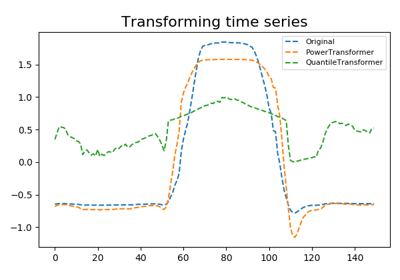Transformers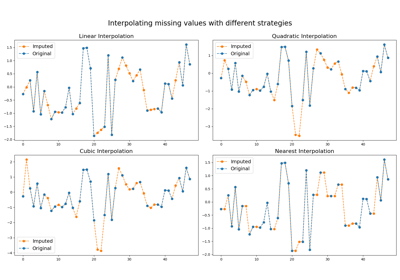ImputerScalers

# Transformation algorithms¶

Transformation algorithms try to capture the most important information from time series using advanced transformation. They can be seen as complex feature extraction algorithms.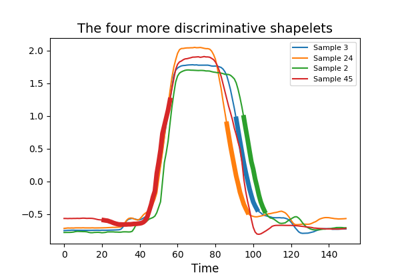Shapelet Transform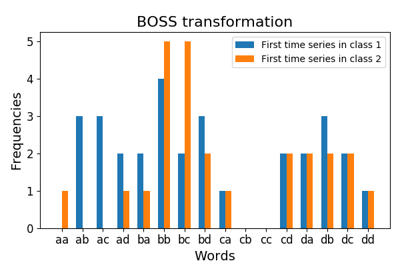Bag-of-SFA Symbols (BOSS)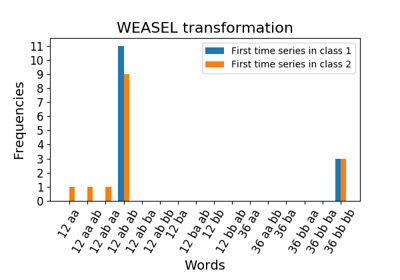Word ExtrAction for time SEries cLassification (WEASEL)

Gallery generated by Sphinx-Gallery